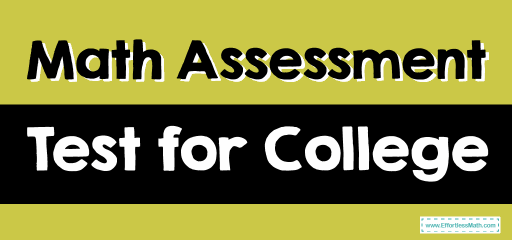# Math Assessment Test for CollegeThe College Placement Test (CPT) provides an assessment of each incoming student’s basic skills level in math, reading, and writing. The result of your Placement Test will determine whether you enter a college algebra course, spend a semester in a Remedial course, or place out of the initial College Algebra class.

One of the main elements of the College Placement Tests is the math test. The result of your exam depends on your understanding of the high school math courses you took, and the accuracy of your Math Placement Test prep.

## The Absolute Best Book to Ace the Accuplacer Math Test

Different versions of the College Placement Test have been made by various states, colleges, and private companies. However, the template and the subjects remain the same in the Math Placement Tests.

• These tests perform by a computer and are untimed.
• You can expect the Basic Math Placement Test to cover pre-algebra and basic arithmetic skills.
• The Algebra Test is generally given as a separate part of the basic test.
• Some incoming students will be given an Advanced Mathematics Placement Test, which consists of geometry, trigonometry, and college algebra.

Three levels have been tested:

• Basic Skills – Basic Math Operation Sequence
• Application – Use basic functions to varying situations
• Analysis – Understanding of more complex mathematical functions and ratios

### Basic Arithmetic and Pre-Algebra Skills

This section assesses the prerequisites of College Algebra I, which includes:

• Integers
• Decimals
• Fractions
• Integer Exponents and Square Roots
• Percentages
• Ratios
• Averages

### Algebra Placement Test

This test focuses on three areas:

• Elementary Algebra
• Coordinate Geometry
• Intermediate Algebra

## The Best Books to Ace the Accuplacer Math Test

### What people say about "Math Assessment Test for College - Effortless Math: We Help Students Learn to LOVE Mathematics"?

No one replied yet.

X
41% OFF

Limited time only!

Save Over 41%

SAVE \$10

It was \$24.99 now it is \$14.99